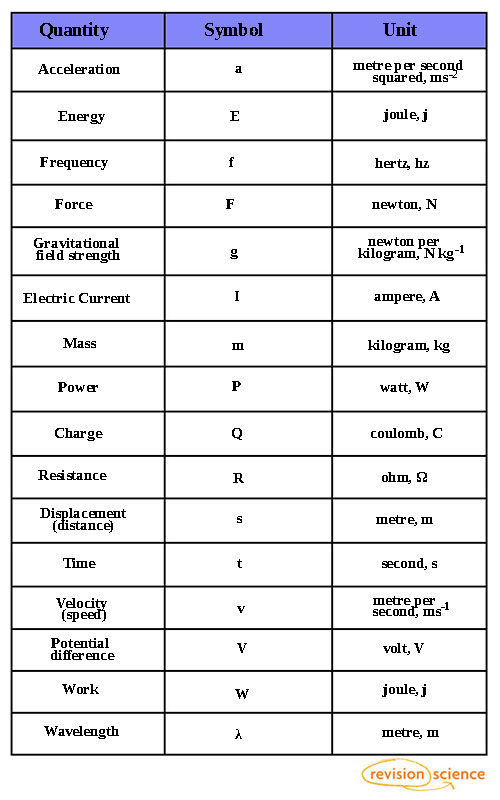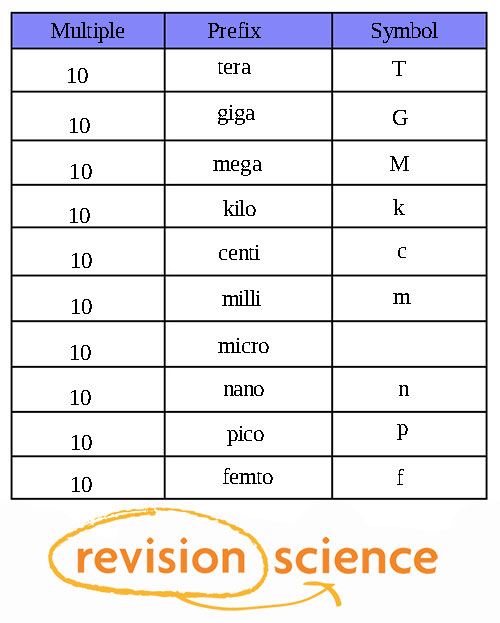## Title

From GCSE to A-Level

At A-Level, you must be able to use standard scientific notation – using conventional symbols and units. You must also be able to write very small and very large numbers in standard form.

The table below shows a variety of scientific quantites and their SI notation.Note: Metres per Second, ms-1, and metres per second squared, ms-2, conform to the rule of indices. Click here to refresh indices.

Standard form allows for very large and small numbers to be written in a more convenient way.

A x 10n where A is a number between 1 and 10 and n is the number of places the decimal point moves.

2100 can be written as 2.1 x 103 and 0.0021 can be written as 2.1 x 10-3  Find out more about standard form on Revision Maths.

Significant figures.

Make sure to give your answers to questions to a sensible number of significant figures.

It may be best to round to the same number of significant figures as the data you're given in the calculation – to the value of least significant figures.

e.g 13 ÷ 3.5 = 3.71428…… = 3.7 (to 1 s.f.)

Large and small numbers

The below table has prefixes and symbols that denote very large and very small quantities.